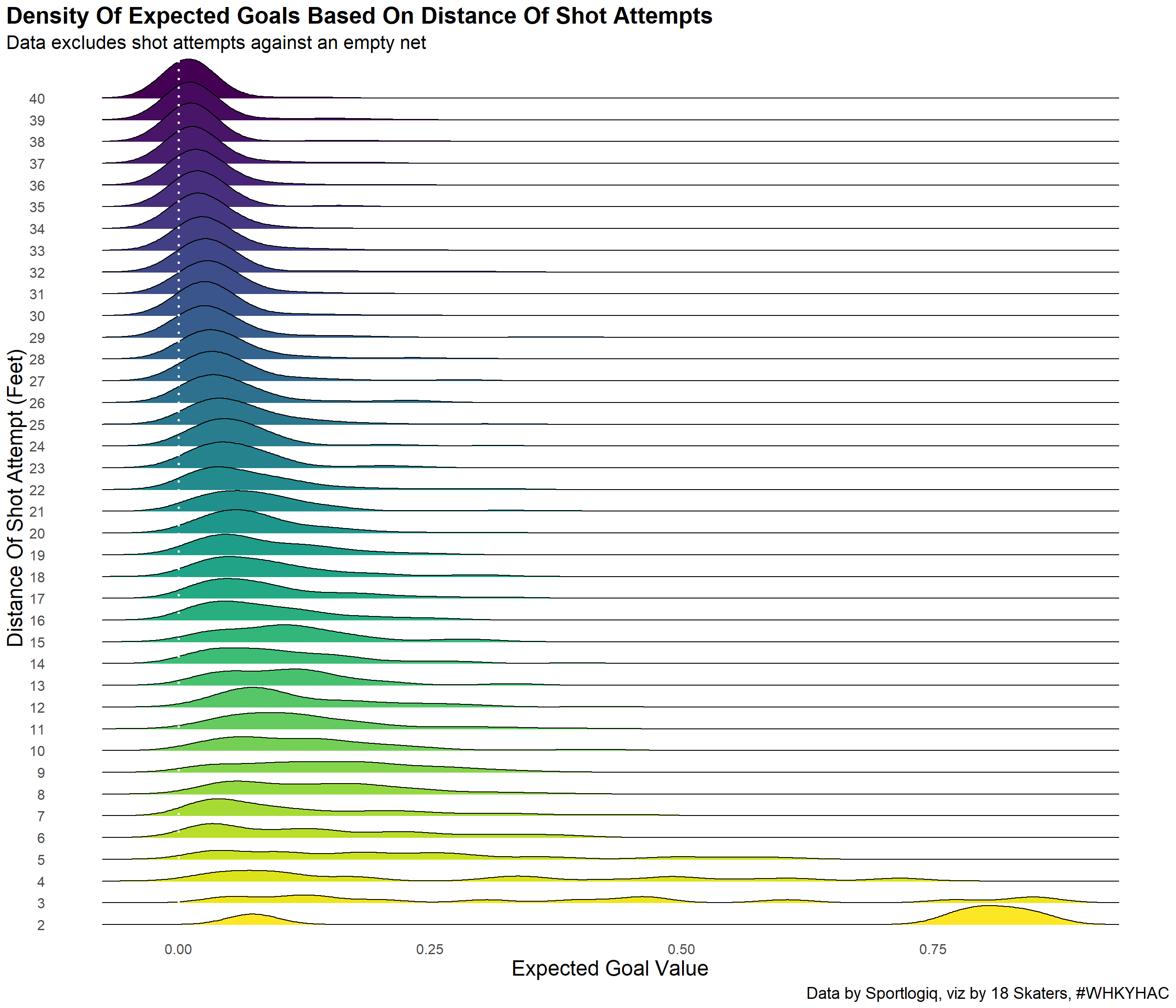### WHKYHAC: Expected Goals Viz

WHKYHAC and Sportlogiq announced a visualization competition using data from the 2023 Season of the Professional Women’s Hockey Players Association. The entry deadline for the competition is July 2, 2023. Prior to that deadline I’ll post some “trial” data visualizations here.

## Today’s Data Viz

The viz in today’s post is really about Sportlogiq’s expected goals model. When I first started working with Sportlogiq’s data I noticed high expected goal values for some of the shot attempts. Sure enough, most of those high xG values were for shot attempts that turned into actual goals. So what was going on?

## Ridgeline Plot Of xGoals And Shot Attempt Distance

When it comes to expected goal models the distance of a shot attempt is hugely important. So I started my investigation into Sportlogiq’s expected goals model by adding distance to the data and plotting the results.The really high expected goal values were for shot attempts taken within a few feet of the center of the net. That makes sense. If the puck is two feet from the center of the net then there isn’t much room for a goalie between the puck and the goal line.

## The Code

The code for this post is pretty basic but I’ll post it anyway. The only “trick” in the code is adding the distance of each shot attempt. It’s not much of a trick, but could be helpful if you’ve never done it before.

``````# LOAD DATA ####################################################################

locale = locale(encoding = "ISO-8859-1"),
show_col_types = FALSE)

# EXPLORE DATA #################################################################

# print(str(raw_pbp_data))

# Players

player_names <- unique(raw_pbp_data\$player)

# Events

event_names <- unique(raw_pbp_data\$eventname)

event_outcomes <- unique(raw_pbp_data\$outcome)

event_types <- unique(raw_pbp_data\$type)

# Strength states

strength_states <- unique(raw_pbp_data\$strengthstate)

# CLEAN AND MANIPULATE DATA (AREAS OF INTEREST ONLY) ###########################

clean_pbp_data <- raw_pbp_data

# Fix name for Kristin O’Neill

clean_pbp_data\$player <- str_replace_all(clean_pbp_data\$player, "\\031", "’")

clean_pbp_data\$game_id <- paste(clean_pbp_data\$game, clean_pbp_data\$date)

clean_pbp_data\$event_id <- seq(1:length(clean_pbp_data\$seasonstage))

# Add empty_net to opposing_goalie variable

clean_pbp_data\$opposing_goalie <- ifelse(
is.na(clean_pbp_data\$opposing_goalie),
"empty_net",
clean_pbp_data\$opposing_goalie)

# Reorganize a little

clean_pbp_data <- select(clean_pbp_data, c(30:31,1:29))

# ADD SHOT ATTEMPT DISTANCE ####################################################

# Euclidean distance formula to determine distance of shot attempt from middle of the net (rounded to a whole number for the purpose of creating a density plot)

clean_pbp_data <- clean_pbp_data %>%
mutate(sa_distance = ifelse(
eventname == "shot",
NA))

# PREP PLOT DATA ###############################################################

# Filter data for shot attempt distance <= 40
# Remove empty nets
# Other plot prep

plot_data <- clean_pbp_data %>%
filter(sa_distance <= 40) %>%
filter(opposing_goalie != "empty_net") %>%
select(teamname,
eventname,
goal,
xg_all_attempts,
sa_distance) %>%
mutate(sa_distance = as.factor(sa_distance))

# PLOT THE DATA ################################################################

plot <- ggplot(data = plot_data,
aes(x = xg_all_attempts,
y = sa_distance,
fill = sa_distance)) +
geom_vline(xintercept = 0,
linetype = 3,
linewidth = 1,
colour = "white") +
theme_minimal() +
theme(panel.grid = element_blank(),
legend.position = "none",
plot.title.position = "plot",
plot.title = element_text(size = 20,
face = "bold"),
plot.subtitle = element_text(size = 16),
axis.title = element_text(size = 18),
plot.caption = element_text(size = 14),
axis.text = element_text(size = 12)) +
scale_fill_viridis_d(direction = -1) +
labs(x = "Expected Goal Value",
y = "Distance Of Shot Attempt (Feet)",
title = "Density Of Expected Goals Based On Distance Of Shot Attempts",
subtitle = "Data excludes shot attempts against an empty net",
caption = "Data by Sportlogiq, viz by 18 Skaters, #WHKYHAC")``````

## The End

That’s it. I’ll post more data visualizations in the days leading up to the July 2 deadline for the Viz Launchpad Competition.

Mark (18 Skaters)